# 很简单的html+js,程序员的浪漫，可以让你小女朋友点开哈。哈哈哈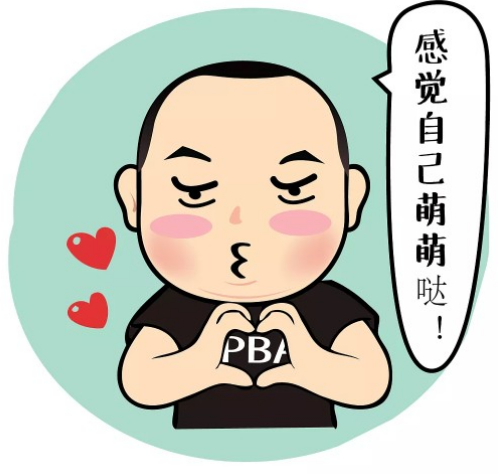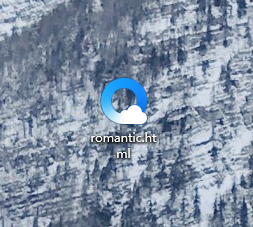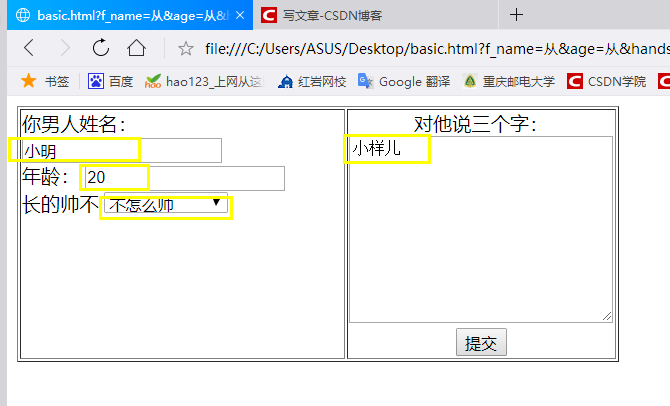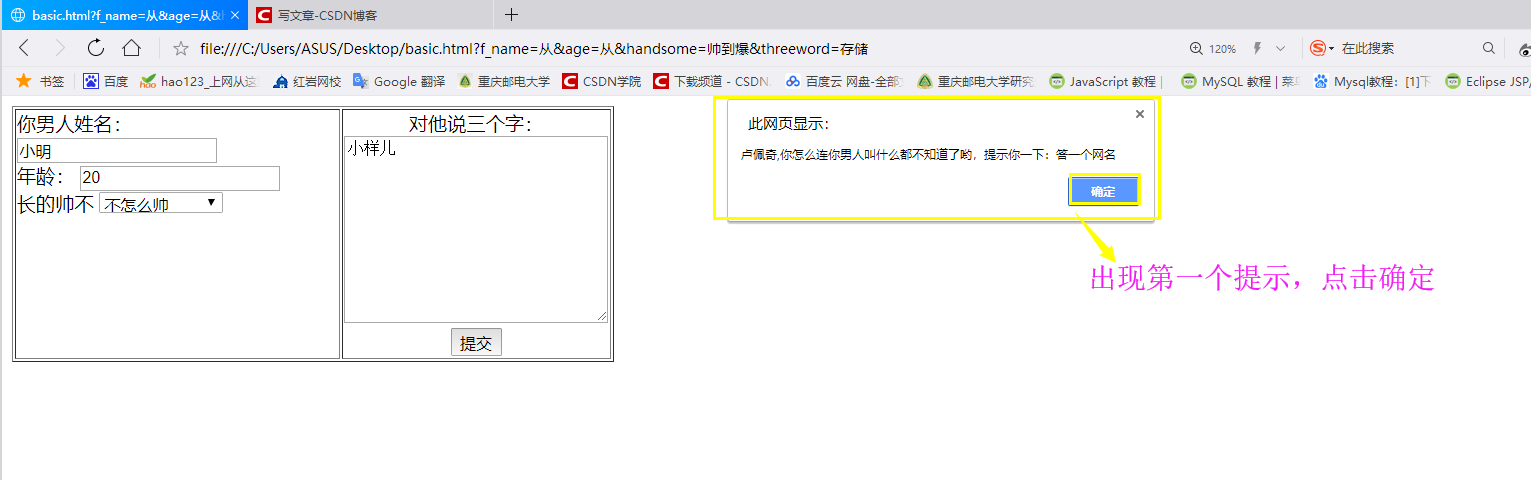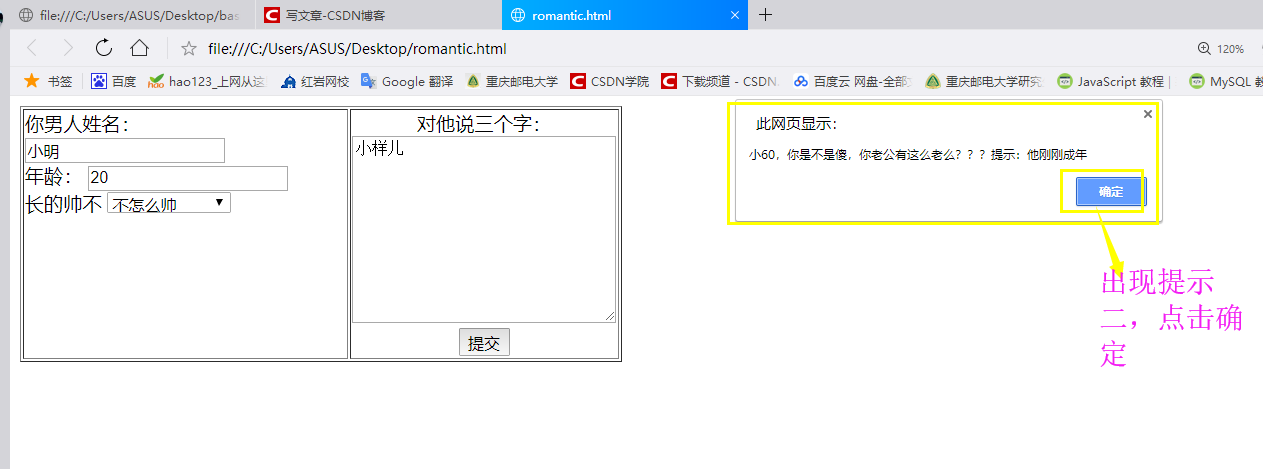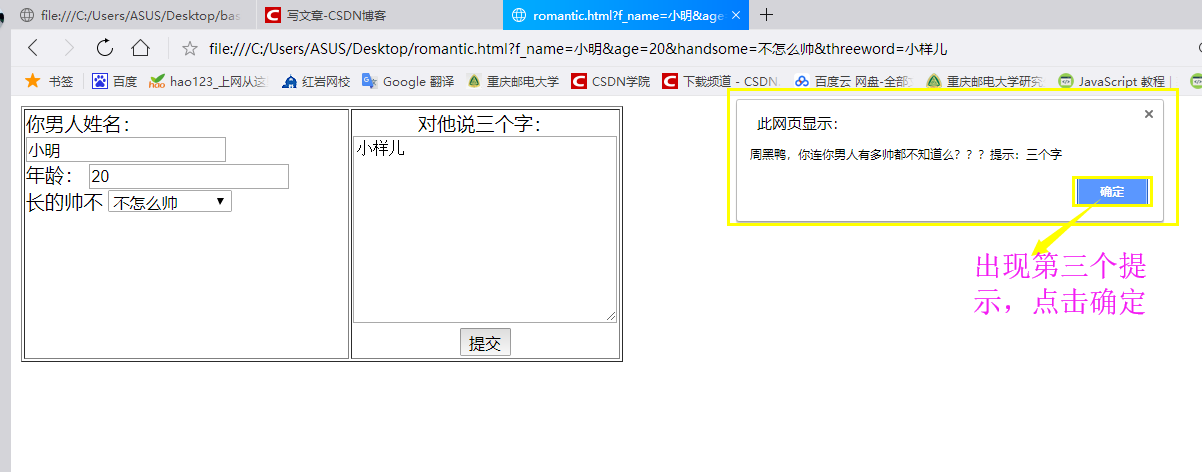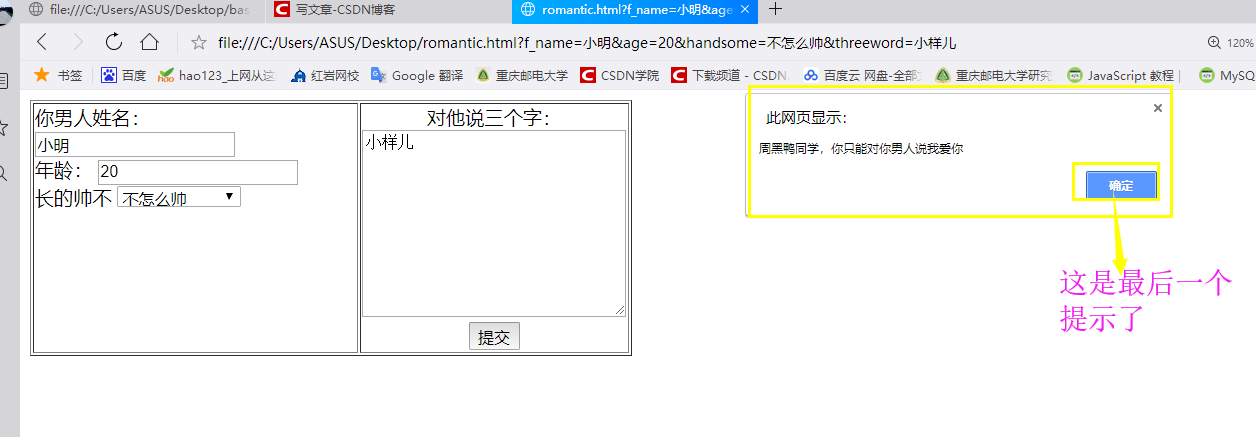<!DOCTYPE html>
<html>
<body>
<form method="get" name="f" id="form1">
<table border="1">
<tr valign="top">
<td>你男人姓名：
<input type="text" id="f_name" name="f_name"><br />

<input type="text" id="age" name="age"><br />

<select name="handsome" id="handsome">
<option value="非常帅">非常帅</option>
<option value="一般帅">一般帅</option>
<option value="有点帅">有点帅</option>
<option value="特别帅">特别帅</option>
<option value="帅到爆">帅到爆</option>
<option value="帅到没法形容">帅到没法形容</option>
<option value="点点帅">点点帅</option>
<option value="不怎么帅">不怎么帅</option>
<option value="不帅">不帅</option>
<option value="没有答案">没有答案</option>
</select>
</td>
<td align="center">对他说三个字：<br />
<textarea rows="10" cols="30" id="threeword" name="threeword"></textarea><br />
<input type="submit" value="提交" onClick="return getMessage();">
</table>
</form>

<script type="text/javascript">
function getMessage()
{
var bian2=f.f_name.value;
if(bian2=="大脸猫"){
alert("名字填写正确")
}else{
alert("卢佩奇,你怎么连你男人叫什么都不知道了哟，提示你一下：答一个网名")
}
var bian3=f.age.value;
if(bian3=="18"){
alert("年龄回答正确")
}else{
alert("小60，你是不是傻，你老公有这么老么？？？提示：他刚刚成年")
}
var bian4=f.handsome.value;
if(bian4=="帅到爆"){
alert("确实是帅到爆")
}else{
alert("周黑鸭，你连你男人有多帅都不知道么？？？提示：三个字")
}
var bian5=f.threeword.value;
if(bian5=="我爱你"){
alert("还好你识相")
}else{
alert("周黑鸭同学，你只能对你男人说我爱你")
}
}
</script>
</body>
</html>